Students who are preparing for the Science exam can download this Tamilnadu State Board Solutions for Class 10th Science Chapter 1 from here for free of cost. These Tamilnadu State Board Textbook Solutions PDF cover all 10th Science Thermal Physics Book Back Questions and Answers.

All these concepts of Chapter 1 Laws of Motion are explained very conceptually by the subject teachers in Tamilnadu State Board Solutions PDF as per the prescribed Syllabus & guidelines. You can download Samacheer Kalvi 10th Science Book Solutions Chapter 1 Laws of Motion State Board Pdf for free from the available links. Go ahead and get Tamilnadu State Board Class 10th Science Solutions of Chapter 1 Laws of Motion.

## Tamilnadu Samacheer Kalvi 10th Science Solutions Chapter 3 Thermal Physics

Kickstart your preparation by using this Tamilnadu State Board Solutions for Class 10th Science Chapter 3 Thermal Physics Questions and Answers and get the max score in the exams. You can cover all the topics of Chapter 3 easily after studying the Tamilnadu State Board Class 10th Science Textbook solutions pdf. Download the Tamilnadu State Board Science Chapter 3 Thermal Physics solutions of Class 10th by accessing the links provided here and ace up your preparation.

### Samacheer Kalvi 10th Science Thermal Physics Textual Solved Problems

Thermal Physics Class 10 Question 1.
A container whose capacity is 70 ml is filled with a liquid up to 50 ml. Then, the liquid in the container is heated. Initially, the level of the liquid falls from 50 ml to 48.5 ml. Then we heat more, the level of the liquid rises to 51.2 ml. Find the apparent and real expansion.
Level of the liquid L1 = 50 ml
Level of the liquid L2 = 48.5 ml
Level of the liquid L3 = 51.2 ml
Apparent expansion = L3 – L1 = 51.2 ml – 50 ml = 1.2 ml
Real expansion = L3 – L1 = 51.2 ml – 48.5 ml = 2.7 ml
So, Real expansion > apparent expansion.

10th Science Thermal Physics Question 2.
Keeping the temperature as constant, gas is compressed four times of its initial pressure. The volume of gas in the container changing from 20 cc (V1 cc) to V2 cc. Find the final volume V2.
Initial pressure (P1) = P
Final Pressure (P2) = 4P
Initial volume (V1) = 20 cc = 20 cm3
Final volume (V2) = ?
Using Boyle’s Law, PV = constant
P1V1 = P2V2
$$\mathrm{V}_{2}=\frac{\mathrm{P}_{1}}{\mathrm{P}_{2}} \times \mathrm{V}_{1}=\frac{\mathrm{P}}{4 \mathrm{P}} \times 20 \mathrm{cm}^{3}$$
V2 = 5 cm3.

### Samacheer Kalvi 10th Science Thermal Physics Textual Evaluation

Thermal Physics Class 10 Book Back Answers Question 1.
The value of universal gas constant ______.
(a) 3.81 mol-1 KJ-1
(b) 8.03 J mol-1 K-1
(c) 1.38 mol-1 KJ-1
(d) 8.31 J mol-1 K-1.
(d) 8.31 J mol-1 K-1.
Hint: Universal gas constant has a fixed value of 8.31 J mol-1 K-1.

Thermal Physics One Mark Questions And Answers Question 2.
If a substance is heated or cooled, the change in mass of that substance is _____.
(a) positive
(b) negative
(c) zero
(d) none of the above.
(c) zero
Hint: Mass is always conserved during any change as the transmission of heat either cooling or heating.

10th Science Thermal Physics Book Back Answers Question 3.
If a substance is heated or cooled, the linear expansion occurs along the axis of:
(a) X or -X
(b) Y or -Y
(c) both (a) and (b)
(d) (a) or (b)
(a) X or -X

Science Solution Class 10 Samacheer Kalvi Question 4.
Temperature is the average _____ of the molecules of a substance?
(a) the difference in K.E and P.E.
(b) the sum of P.E and K.E.
(c) the difference in T.E and P.E.
(d) the difference in K.E and T.E.
(c) the difference in T.E and P.E.
Hint: As total energy of a substance is equal to the sum of the K.E, and P.E of atoms (or) molecules
Temperature is equal to the average KE of molecules
K.E = T.E – P.E.

Samacheer Kalvi 10th Science Solution Book Question 5.
In the Given diagram, the possible direction of heat energy transformation is _____.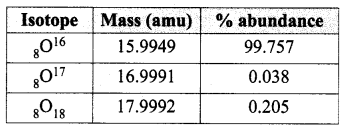(a) A ← B, A ← C, B ← C
(b) A → B, A → C, B → C
(c) A → B, A ← C, B → C
(d) A ← B, A → C, B ← C.
(a) A ← B, A ← C, B ← C
Hint: Heat energy flows from a system of higher to lower temperature.
So the heat will flow towards lowest temperature A. i.e., B → A & C → A as the temperature of C is more than B.
The heat will flow C → B.

II. Fill in the blanks

Class 10 Science Chapter 3 Answers Question 1.
The value of Avogadro number _____.
6.023 × 1023 / mol.

Question 2.
The temperature and heat are _____ quantities.
Scalar.

Question 3.
One calorie is the amount of heat energy required to raise the temperature of _____ of water through ____.
1 gram, 1°C.

Question 4.
According to Boyle’s law, the shape of the graph between pressure and reciprocal of the volume is ______.
Straight line.

III. State whether the following statements are true or false. if false explain why?

Question 1.
For a given heat in liquid, the apparent expansion is more than that of real expansion.
False.
Correct Statement: When the liquid is heated in a container, first heat is gained by container which expands and level of liquid goes down, but immediately liquid receives the heat from container so apparent expansion is liquid.
Real expansion = Expansion of container + Apparent expansion

Question 2.
Thermal energy always flows from a system at a higher temperature to a system at a lower temperature.
True.

Question 3.
According to Charles’s law, at constant pressure, the temperature is inversely proportional to volume.
False.
Correct Statement: According to Charle’s law at constant pressure (P), the volume of gas is directly proportional to absolute temperature.
V ∝ T
(or)
T ∝ V.

IV. Match the items in column-I to the items in column-II

Question 1.

 Column-I Column-II 1. Linear expansion (a) change in volume 2. Superficial expansion (b) hot body to cold body 3. Cubical expansion (c) 1.381 × 10-23 JK-1 4. Heat transformation (d) change in length 5. Boltzmann constant (e) change in an area

1. (d) change in length
2. (e) change in the area
3. (a) change in volume
4. (b) hot body to cold body
5. (c) 1.381 × 10-23 JK-1

V. Assertion and Reason Type Questions

(a) Both the assertion and the reason are true and the reason is the correct explanation of the assertion.
(b) Both the assertion and the reason are true but the reason is not the correct explanation of the assertion.
(c) Assertion is true but the reason is false.
(d) Assertion is false but the reason is true.

Question 1.
Assertion: There are no effects on other ends when the end of the rod is only heated.
Reason: Heat always flows from a region of lower temperature to higher temperature of the rod.
(c) The assertion is true but the reason is false.
Correct Reason: Heat always flows from a region of higher temperature to lower the temperature of the rod.

Question 2.
Assertion: Gas is highly compressible than solid and liquid
Reason: Interatomic or intermolecular distance in the gas is comparably high.
(a) Both the assertion and the reason are true and the reason is the correct explanation of the assertion.

Question 1.
Define one calorie.
One calorie is defined as the amount of heat energy required to raise the temperature of 1 gram of water through 1°C.

Question 2.
Distinguish between linear, cubical and superficial expansion.

 Linear expansion Cubical expansion Superficial expansion When a body is heated or cooled, the length of the body changes due to change in its temperature. If there is an increase in the volume of a solid body due to heating, it is called as cubical expansion. If there is an increase in the area of a solid object due to heating, then the expansion is called superficial expansion. The ratio of increase in the length of the body per degree rise in temperature to its unit length. The ratio of increase in the volume of the body per degree rise in temperature to its unit volume is called a coefficient of cubical expansion. The ratio of increase in the area of the body per degree rise in temperature to its unit area is called a coefficient of superficial expansion.

Question 3.
What is co-efficient of cubical expansion?
The ratio of increase in volume of the body per degree rise in temperature to its unit volume is called as coefficient of cubical expansion. This is also measured in K-1.

Question 4.
State Boyle’s law.
When the temperature of a gas is kept constant, the volume of a fixed mass of gas is inversely proportional to its pressure.
$$\mathrm{P} \propto \frac{1}{\mathrm{V}}$$.

Question 5.
State-the law of volume.
According to this law, When the pressure of gas is kept constant, the volume of a gas is directly proportional to the temperature of the gas.
V ∝ T
(OR)
$$\frac{V}{T}$$ = constant

Question 6.
Distinguish between ideal gas and real gas.

 Ideal Gas Real Gas If the atoms or molecules of gas do not interact with each other then it is called as an ideal or perfect gas. If the atoms or molecules of a gases interact with each other with a definite amount of intermolecular (or) interatomic force, then it is called as real gas. At very high temperature (or) low pressure, a real gas behaves as an ideal gas, because there is no interatomic (or) Intermolecular force of attraction. At very high temperature (or) low pressure, the interatomic (or) Intermolecular forces of attraction are weak in an ideal gas.

Question 7.
What is the co – efficient of real expansion?
Coefficient of real expansion is defined as the ratio of the true rise in the volume of the liquid per degree rise in temperature to its unit volume.
The SI unit of coefficient of real expansion is K-1.

Question 8.
What is co-efficient of apparent expansion?
Coefficient of apparent expansion is defined as the ratio of the apparent rise in the volume of the liquid per degree rise in temperature to its unit volume. The SI unit of coefficient of apparent expansion is K-1.

VII. Numerical Problems

Question 1.
Find the final temperature of a copper rod. Whose area of cross-section changes from 10 m2 to 11 m2 due to heating. The copper rod is initially kept at 90 K. (Coefficient of superficial expansion is 0.0021 K)?
Solution:
Given:
Area of copper rod A0 = A1 = 10 m2
Changes of area cross-section ∆A = A2 – A1 = 11 – 10 = 1 m2
Initial temperature t1 = 90 K
∆T = t2 – t1 = t2 – 90
Coefficient of superficial expansion αA = 0.0021 / K Then t2 = ?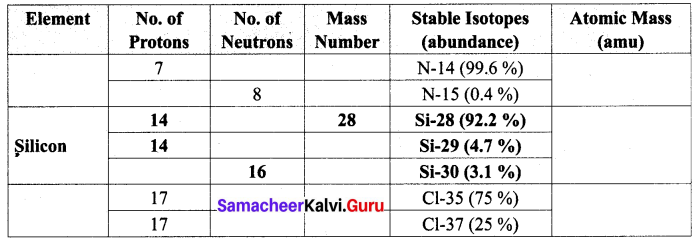Question 2.
Calculate the coefficient of cubical expansion of a zinc bar. Whose volume is increased 0. 25 m3 from 0.3 m3 due to the change in its temperature of 50 K?
Solution:
Given: αv of Zn bar
Volume of zinc bar V0 = V1 = 0.3 m3
Change in volume ∆V = V2 – V1 = 0.25 – 0.30 = 0.05 m3
Change in temperature ∆T = 50K
Coefficient of cubical expansion αv = ?
$$\alpha_{\mathrm{v}}=\frac{\Delta \mathrm{V}}{\mathrm{V}_{\mathrm{o}} \Delta \mathrm{T}}=\frac{0.05}{0.3 \times 50}=\frac{0.05}{15}$$
αv = 0.0033 K-1

Question 1.
Derive the ideal gas equation.
The ideal gas equation is an equation, which relates all the properties of an ideal gas. An ideal gas obeys Boyle’s law and Charles’ law and Avogadro’s law.
According to Boyle’s law,
PV = constant ……. (1)
According to Charles’s law,
$$\frac { V }{ T }$$ = constant ……. (2)
$$\frac { V }{ n }$$ = constant ……. (3)
After combining equations (1), (2) and (3), you can get the following equation.
$$\frac { PV }{ nT }$$ = constant ……. (4)
The above relation is called the combined law of gases. If you consider a gas, which contains μ moles of the gas, the number of atoms contained will be equal to p times the Avogadro number, NA
i.e. n = μNA ……. (5)
Using equation (5), equation (4) can be written as
PV/ μNAT = constant
The value of the constant in the above equation is taken to be kB, which is called as Boltzmann constant (1.38 × 10-23 JK-1).
Hence, we have the following equation:
PV / μNAT = kB
PV = μNAkBT
Here, μNAkB = R, which is termed as universal gas constant whose value is 8.31 J mol-1 K-1.
PV = RT ……. (6)
The ideal gas equation is also called an equation of state because it gives the relation between the state variables and it is used to describe the state of any gas.

Question 2.
Explain the experiment of measuring the real and apparent expansion of a liquid with a neat diagram.
(i) To start with, the liquid whose real and apparent expansion is to be determined is poured in a container up to a level. Mark this level as L1.
(ii) Now, heat the container and the liquid using a burner as shown in the figure Initially, the container receives the thermal energy and it expands.
(iii) As a result, the volume of the liquid appears to have reduced. Mark this reduced level of liquid as L2.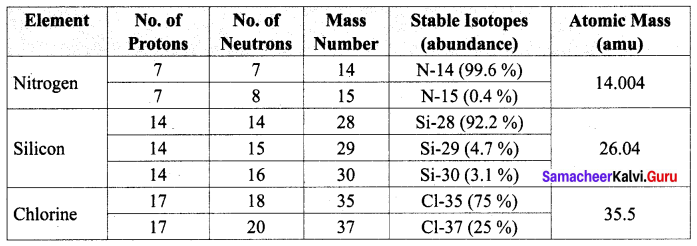On further heating, the thermal energy supplied to the liquid through the container results in the expansion of the liquid. Hence, the level of liquid rises to L3. Now, the difference between the levels L1 and L3 is called apparent expansion, and the difference between the levels L2 and L3 is called real expansion. The real expansion is always more than that of apparent expansion.
Real expansion = L3 – L2
Apparent expansion = L3 – L1.

IX. HOT Questions

Question 1.
If you keep ice at 0°C and water at 0°C in either of your hands, in which hand you will feel more chillness? Why?
The hand having ice at 0°C will feel more chillness than of 0°C water.
Reason: The 0°C ice takes more heat from hand equal to mL, to convert 0°C Ice water, 0°C as compared to 0°C water of same mass. Hence m & L are the mass and latent heat of fusion of water respectively.

### Samacheer Kalvi 10th Science Thermal Physics Additional Questions

Question 1.
At constant temperature, the volume of a given mass of gas is inversely proportional to its pressure is _____.
(a) Charle’s law
(b) Boyle’s law
(d) None of these.
(b) Boyle’s law
Hint: At constant temperature, only Boyle’s law is there.

Question 2.
The value of equivalent to 0°C on the absolute scale is:
(a) – 273 K
(b) OK
(c) 273 K
(d) 373 K
(c) 273 K

Question 3.
At constant pressure, the volume of a given mass of gas is proportional to _____.
(a) temperature
(b) atmospheric pressure
(c) absolute temperature
(d) none of these.
(a) temperature
Hint: Charle’s law gives the relation between V & T at constant pressure.

Question 4.
The value of absolute zero temperature is:
(a) 273° C
(b) 100° C
(c) 0° C
(d) -273° K
(d) -273° K

Question 5.
The ratio of change in dimension to the original dimension per degree kelvin change in temperature is _____.
(a) coefficient of linear expansion
(b) coefficient of cubical expansion
(c) coefficient of thermal expansion
(d) coefficient of areal expansion.
(c) coefficient of thermal expansion
Hint: Coefficient of linear expansion
$$=\frac{\text { Change in dimension }}{\text { Original dimension } \times \Delta \mathrm{T}}$$.

Question 6.
The relation between Fahrenheit and Kelvin scales of temperature is:
(a) K = F × $$\frac{5}{9}$$
(b) K = F – C
(c) K = (F + 460) × $$\frac{9}{5}$$
(d) K = (F + 460) × $$\frac{5}{9}$$
(d) K = (F + 460) × $$\frac{5}{9}$$

Question 7.
Increase in area per unit area at 0°C per degree rise in temperature is _____.
(a) coefficient of linear expansion.
(b) coefficient of superficial expansion.
(c) coefficient of cubical expansion.
(d) none of these.
(b) coefficient of superficial expansion.
Hint: As the superficial expansion is directly proportional to the original area of sheet and rise in temperature.

Question 8.
Coefficient of linear expansion depends upon _____.
(a) pressure
(b) volume
(c) nature of material
(d) none of these.
(c) nature of material
Hint: α of a material depend on nature of material of rod.

Question 9.
If the temperature is the effect then ……… is the cause.
(a) Energy
(b) Work
(c) Heat
(d) Mass
(c) Heat

Question 10.
For the measurement heat in the body following one of these is used _____.
(a) thermometer
(b) calorimeter
(c) pressure gauge
(d) multimeter.
(b) calorimeter
Hint: Heat of a body can be measured with the help of calorimeter.

Question 11.
Transfer of heat from the hot end to cold end with out-migration of a molecule of solid is called _____.
(a) convection
(b) conduction
(d) none of these.
(b) conduction
Hint: Heat flows from without migration of particle is conduction.

Question 12.
Amount of heat required to convert 10 g of ice at 0°C, into water at same temperature is:
(a) 3000 J
(b) 336 J
(c) 300 J
(d) 3360 J
(b) 336 J

Question 13.
Process of transfer of heat in the form of electromagnetic wave (light) for which material medium not necessary is _____.
(a) conduction
(c) convection
(d) none of these.
Hint: Heat can flow even in a vacuum (without medium) by radiation of electromagnetic wave.

Question 14.
When ice melts its volume:
(a) Increases
(b) Changes
(c) Decreases
(d) Remains same
(c) Decreases

Question 15.
When 1 kg of water at 100°C is mixed with 1 kg of water at 65°C, the temperature of the mixture is:
(a) Less than 100°C
(b) Less 100°C
(c) Equal to 100°C
(d) Less than 100°C but more than 65°C
(d) Less than 100°C but more than 65°C

II. Fill in the Blanks

Question 1.
_____ is the amount of heat required to raise the temperature of ____ water through 1 kelvin.
one kilocalorie, one kg.

Question 2.
A thermometer is used to measure _____.
Temperature.

Question 3.
In _____ scale of temperature no negative value of temperature.
Kelvin.

Question 4.
_____ is the substance whose physical property is utilised for measuring temperature.
Thermometric substance.

Question 5.
_____ is that temperature attained by two bodies when they are brought in thermal contact with each other are in thermal equilibrium, is attained.
Equilibrium Temperature.

Question 6.
The lowest temperature attainable according to Charle’s law is _____.
-273°C.

Question 7.
The P.E. of molecules in ideal gas to _____.
Zero.

Question 8.
The nature of graph of P versus $$\left(\frac{1}{V}\right)$$ for the given mass of gas at constant temperature is _____.
Straight line.

Question 9.
As K.E. of the gas increases then the _____ increases.
Temperature.

Question 10.
As pressure on a gas increases the P.E. between molecules of gas _____.
decreases.

Question 11.
The relation between N (no. of molecules), P, V & T is _____.
$$\mathrm{N}=\frac{\mathrm{K}_{\mathrm{B}} \mathrm{T}}{\mathrm{PV}}$$.

Question 12.
H2 gas can behave like an ideal gas at ______ pressure and _____ temperature.
low, high.

III. True or False. If false correct it.

Question 1.
According to Avogadro’s law, equal volumes of all gases under same pressure have equal numbers of molecules.
True.

Question 2.
Boltzmann’s constant k is equal to the product of universal gas constant R and Avogadro number (NA)?
False.
Correct Statement: $$\mathrm{K}_{\mathrm{B}}=\frac{\mathrm{R}}{\mathrm{N}_{\mathrm{A}}}$$

Question 3.
Number of molecules N in gas is equal to the product of the number of mole (n) of gas and Avogadro’s number (NA)?
True.

Question 4.
A gas which obeys the relation PV = nRT at all temperature and pressure is called real gas.
True.

Question 5.
If Q calorie heat is produced by spending mechanical work W then the mechanical equivalent of heat J = W × Q?
False.
Correct Statement: $$\mathrm{J}=\frac{\mathrm{W}}{\mathrm{Q}}$$.

Question 6.
The S.I. unit of coefficient of thermal expansion is per kelvin.
True.

Question 7.
The real expansion of a liquid is equal to the difference between apparent expansion and expansion of the vessel.
False.
Correct Statement: Real expansion = Apparent expansion + expansion of vessel.

IV. Match the following

Question 1.

 1. Heat (a) Joule 2. Thermometer (b) Heating 3. The SI unit of heat (c) Temperature 4. Cool a cup of coffee, adding cold milk (d) Energy 5. gain by heat by a body (e) thermal equilibrium

1. (d) Energy
2. (c) Temperature
3. (a) Joule
4. (e) thermal equilibrium
5. (b) Heating

Question 2.

 1. Boyle’s Law (a) $$\mathrm{K}_{\mathrm{B}}=\frac{\mathrm{R}}{n}$$ 2. Absolute Scale (b) V ∝ t 3. Charle’s Law (c) $$\mathrm{P} \propto \frac{1}{\mathrm{V}}$$ 4. Boltzmann Constant (d) V ∝ n 5. Avogadro’s Law (e) 0 K (zero kelvin)

1. (c) $$\mathrm{P} \propto \frac{1}{\mathrm{V}}$$
2. (e) 0 K (zero kelvin)
3. (b) V ∝ t
4. (a) $$\mathrm{K}_{\mathrm{B}}=\frac{\mathrm{R}}{n}$$
5. (d) V ∝ n

V. Assertion and Reasoning Type Questions

Mark the correct choice as
(a) Both the assertion and the reason are true and the reason is the correct explanation of assertion.
(b) Both the assertion and the reason are true but the reason is not the correct explanation of the assertion.
(c) Assertion is true but the reason is false.
(d) Assertion is false but the reason is true.

Question 1.
Assertion: Good conductors of heat are also a good conductor of electricity and vice-versa
Reason: Mainly electrons are responsible for there conduction.
(a) Both assertion and reason are true and reason is the correct explanation of assertion.

Question 2.
Assertion: Air at some distance above the fire is hotter than the same distance sidewise.
Reason: Air surrounding the fire carries heat upward due to conventional current.
(b) Both assertion and reason are true but reason is not the correct explanation of assertion.
Explanation: Quantity of heat by hot air due to hot conventional current upward is larger by heat side wise due to radiation.

Question 3.
Assertion: As the temperature of a gas increases the K.E of molecules increases, but P.E. decreases.
Reason: Due to the law of conservation of energy.
(a) Both assertion and reason are true and reason is the correct explanation of assertion.

Question 4.
Assertion: Real expansion in liquids is always larger than apparent expansion.
Reason: Vessel of liquid expands 1st then the expansion of liquid in the vessel.
(a) Both assertion and reason are true and reason is the correct explanation of assertion.

Question 5.
Assertion: There are no effects on other ends when one end of the rod is only heated.
Reason: Heat always flows from a region of higher temperature to lower the temperature of the rod.
(d) An assertion is false but the reason is true.
Explanation: There are small effects forms on another end when one end of the rod is only heated.

Question 1.
What do you mean by triple point of water? Why it is unique?
It is the temperature at which the three phases of water and gas of water are equally stable.

Question 2.
State the commonly used units of Temperature.
The commonly used units of temperature are Kelvin, degree Celsius (°C) and degree Fahrenheit (°F).

Question 3.
Can the temperature of a body be negative on the kelvin scale?
The temperature of a body can never be negative on kelvin. Because the lowest temperature on the Kelvin scale is 0 kelvin.

Question 4.
Do all solids expand on heating? If not give an example.
All solid bodies do not expand on heating, semiconductor and camphor contracts on heating.

Question 5.
When are two physical bodies said to be in thermal equilibrium?
Two or more physical systems or bodies are said to be in thermal equilibrium if there is no net flow of thermal energy between the systems.

Question 6.
Why does the solid expand on heating?
When heat energy is supplied to a substance the E of molecule or atoms increases so the average distance between then increases. So the volume of matter (solid, liquid, gas) increases.

Question 7.
Why does a small gap is left between the iron rails on a railway track?
Either due to temperature variation in summer and winter or due to frictional force between the wheel of train and rail, when the temperature increases the length of rail will increase and may bend in summer and the train may get derailed.

Question 8.
What is meant by Thermal energy?
Thermal energy is a form of energy which is transferred between any two bodies due to the difference in their temperatures. Thermal energy is also known as ‘heat energy’ or simply ‘heat’.

Question 9.
Cooking gas containers are kept in lorry moving with uniform speed what will be the effect on the temperature of gas molecules?
If the lorry is moving with uniform speed the speed of cooking gas molecules will also be uniform and the collisions will be least or loss of K.E will be least in turn increase in temperature will be less. If the lorry is not moving with uniform the cylinders and molecules collide more and more and their temperature may increase and can cause the fire in gas.

Question 10.
Define one Kilocalorie.
One kilocalorie is defined as the amount of heat energy required to rise the temperature of 1 kilogram of water through 1°C.

Question 11.
At what temperature does all molecular motion cease? Explain.
If molecular motion ceases then KE become zero and absolute scale of temperature is directly proportional to KE
T ∝ KE
µ KE = 0, T = 0 K
So at 0 – kelvin temperature motion of all molecules ceases.

Question 12.
Mention different types of expansion.

1. Linear expansion
2. Superficial expansion
3. Cubical expansion

Question 13.
Differentiate between conduction, convection and radiation.

 Conduction Convection Radiation Material medium is required. Material medium is required. Material medium is required. It is due to the temperature difference. It is due to density difference. It occurs from all bodies above 0 K. Heat flows from higher to lower temperature. Heat flows from lower to higher density. Heat flows from higher to lower temperature. It heats up the medium. It also heats up the medium. It does not heat up the medium. It is a slow process. It is also a slow process. It propagates with speed of light. It does not obey the laws of refraction and reflection. It also does not obey the laws of refraction and reflection. It obeys the law of refraction and reflection.

Question 14.
Define co-efficient of linear expansion. State its unit.
The ratio of increase in length of the body per degree rise in temperature to its unit length is called as the coefficient of linear expansion. The SI unit of Coefficient of Linear expansion is K-1.

VII. Numerical Problems

Question 1.
At what temperature the value of Celcius and Fahrenheit scale coincide.
Solution: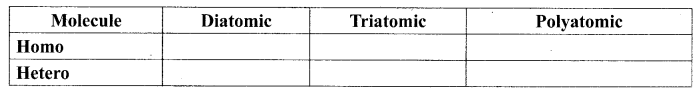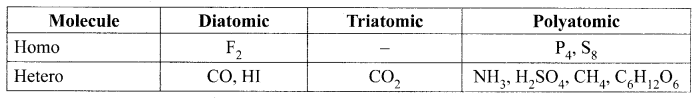Question 2.
A constant volume thermometer using the gas reads a pressure of 1.75 × 104 Pa at normal freezing of water and reads 2.39 × 104 pa at normal boiling point of water. Obtained from the observation the temperature of absolute zero on the Celcius scale.
Solution:
Let temperature on Kelvin scale of the freezing point of water = T1K = 2.39 × 104 Pa
Let temperature on Kelvin scale of a boiling point of water = T2K = 1.75 × 104 Pa
As volume is constant so by universal gas law.
PV = nRT as volume and mass of gas in thermometer constant,
so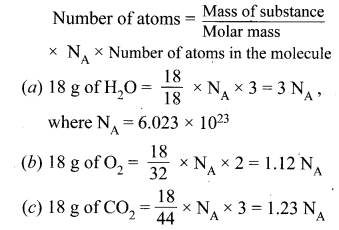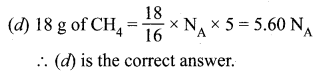Question 3.
Convert 323 K to °C.
T = 323 K
T°C = 323 – 273
= 50°C
∴ 323 K = 50°C

Question 4.
If the volume of a block of metal changes by 12% when it is heated by 30°C. What is the coefficient of linear expansion, superficial expansion?
Solution: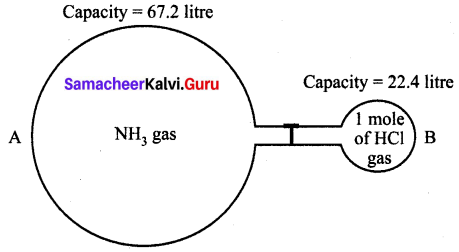Question 5.
If the volume of a metallic block increases by 0.12% when it is heated through 20°C, what is the coefficient of cubical expansion.
$$\frac{ΔV}{V}$$ = 0.12% = $$\frac{0.12}{100}$$
Change in temperature ΔT = 20°C
Coefficient of cubical expansion
αV = $$\frac{ΔV}{VΔT}$$
= $$\frac{0.12}{100×20}$$
αV = 6 × 10-6/°C

Question 1.
What are the characteristic feature of transfer of heat energy?

1. Heat always flows from a system at higher temperature to a system at lower temperature.
2. The mass of a system is not altered when it is heated or cooled.
3. For any exchange of heat, the heat gained by the cold system is equal to heat lost by the hot system. Heat gained = Heat lost

Question 2.
In the upper atmosphere, the kinetic temperature of the air is of the order of 1000 K even then one feels severe cold in these places. Why?
As we go at higher altitude the density of air decreases. So distances between molecules increases due to less number of collisions between them. Due to above reason speed of air molecules, the kinetic temperature is of order 1000 K but due to higher altitude, the temperature decreases as the radiation from earth takes longer distance.

IX. Conceptional or Hot Questions.

Question 1.
The earth without its atmosphere would be inhospitably cold why?
The earth without its atmosphere would be inhospitably cold.

Question 2.
Why does the pendulum clock run slow in summer and fast in winter?
As we know that time period of pendulum clock $$\mathrm{T}=2 \pi \sqrt{\frac{\mathrm{L}}{\mathrm{g}}}$$ at a place g and 2π are constant. so $$\mathrm{T} \alpha \sqrt{\mathrm{L}}$$
The length of pendulum in summer increases so the time period of a pendulum is increased. So pendulum takes more time to complete one oscillation and the clock becomes slow in summer. Vice – versa in winter it becomes fast as a time period of a pendulum is decreased due to a decrease in length of a pendulum.

Question 3.
Can you boil water in a paper cup? Explain.
Yes, we can boil water in the paper cup due to the following reason.

• Most type of paper bums at about 230°C.
• Water can pass at the bottom through pores of the paper.
• Hot water rises up due to hot conventional current and replaces the cold water.
• Water boils at 100°C.

The heat of bums warms the water which rises up in a cup, by replacing cold water so heat does not heat up the paper. So water boils in a cup of paper.

Question 4.
The surroundings above a fire is hotter than the surroundings below the fire. Why?